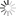Pakistan

SCHOLARLY PAPERS

Rank 5,688

SSRN RANKINGS

7,528

SSRN CITATIONS
Rank 40,543

SSRN RANKINGS

Top 40,543

in Total Papers Citations

0

CROSSREF CITATIONS

13

Ideas:
“  I am working on semi-analytic solution to Fokker-Planck equation.  ”

Scholarly Papers (12)

1.

Solution of Stochastic Volatility Models Using Variance Transition Probabilities and Path Integrals

Number of pages: 25 Posted: 20 Sep 2012 Last Revised: 13 Nov 2012
Ahsan Amin
Infiniti Derivatives Technologies

Abstract:stochastic volatility, Fokker-Planck, Forward Kolmogorov, path integral, transition probabilities

2.

Stochastic Calculus of Standard Deviations: An Introduction

Number of pages: 29 Posted: 10 Oct 2013
Ahsan Amin
Infiniti Derivatives Technologies

Abstract:Stochastic Calculus, Density of SDEs, Variance of SDEs, Stochastic Integrals

3.

Calibration, Simulation and Hedging in a Heston Libor Market Model with Stochastic Basis

Number of pages: 26 Posted: 08 Nov 2010
Ahsan Amin
Infiniti Derivatives Technologies
Citation 4

Abstract:LIBOR Market Model, Forwarding Curve, Discount Curve, Swaption Pricing, Calibration, Basis Greeks, Stochastic Volatility, Stochastic Basis, Forward Curves

4.

Multi-Factor Cross Currency Libor Market Models: Implementation, Calibration and Examples

Number of pages: 14 Posted: 10 Aug 2008
Ahsan Amin
Infiniti Derivatives Technologies
Citation 13

Abstract:cross-currency LIBOR market models, PRDC, Bermudan callable derivatives, Least square monte carlo

5.

Hybrid Equity, FX, and Interest Rate Models with Stochastic Volatility and Jump Diffusion

Number of pages: 15 Posted: 02 Jan 2011 Last Revised: 07 Feb 2011
Ahsan Amin
Infiniti Derivatives Technologies

Abstract:Foreign-Exchange (FX);Equities, Stochastic Volatility, Heston Model, Jump Diffusion, Stochastic Interest Rates, Interest Rate Smile, Forward Characteristic Function, Hybrids, Affine Diffusion, Efficient Calibration, BGM, LIBOR Market Model

6.

Analytic Solution of Convection-Diffusion Partial Differential Equations Using Girsanov Theorem, Feynman-Kac Formula and Brownian Bridge

Number of pages: 15 Posted: 21 Apr 2013
Ahsan Amin
Infiniti Derivatives Technologies

Abstract:Convection-Diffusion PDEs, Girsanov, Feynman-Kac Formula, Derivatives Pricing

7.

On the General Solution of Initial Value Problems of Ordinary Differential Equations Using the Method of Iterated Integrals

Number of pages: 32 Posted: 26 Nov 2016 Last Revised: 25 Jan 2017
Ahsan Amin
Infiniti Derivatives Technologies

Abstract:Ordinary Differential Equations

8.

Calculating Delta Greeks of Callable Exotics in the LMM: A New Approach

Number of pages: 19 Posted: 28 Nov 2009 Last Revised: 24 Feb 2010
Ahsan Amin
Infiniti Derivatives Technologies

Abstract:Delta Greeks, Sensitivities, Callable LIBOR exotics, LMM, LIBOR Market Model, Regression methods

9.

Pricing Bermudan Callable Derivatives with Default, Collateral Margining, Funding and Investment Costs

Number of pages: 25 Posted: 16 May 2012
Ahsan Amin
Infiniti Derivatives Technologies

Abstract:two curve LMM, funding cost, cost of funding, bilateral counterparty risk, credit valuation adjustment, collateral modeling, margining cost, close-out, re-hypothecation, default correlation

10.

Solution of Convection-Diffusion Partial Differential Equations Using Girsanov Theorem and Conditional Brownian Local Time

Number of pages: 8 Posted: 22 Feb 2013
Ahsan Amin
Infiniti Derivatives Technologies

Abstract:Convection Diffusion Partial Differential Equations, Kolmogorov PDE, Brownian Local Time, Stochastic Volatility, Girsanov Theorem

11.

Method of Iterated Integrals for Solution of Stochastic Integrals with Applications to Monte Carlo Simulation of Stochastic Differential Equations.

Number of pages: 27 Posted: 14 Mar 2018
Ahsan Amin
Infiniti Derivatives Technologies

Abstract:Monte Carlo, Stochastic Differential Equations, Simulation, Stochastic Integrals

12.

Calculation of Transition Probabilities of SDEs Only from the Knowledge of Marginal Probabilities Using the CDF-Equivalent Brownian Motion Method

Number of pages: 12 Posted: 17 Feb 2018
Ahsan Amin
Infiniti Derivatives Technologies